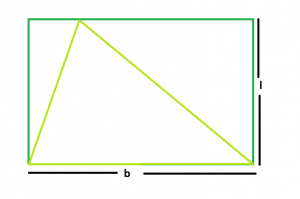Open in App
Not now

# Area of largest triangle that can be inscribed within a rectangle

• Difficulty Level : Basic
• Last Updated : 28 Aug, 2022

Given a rectangle of lengthand breadth. The task is to find the area of the biggest triangle that can be inscribed in it.
Examples

```Input: L = 5, B = 4
Output: 10

Input: L = 3, B = 2
Output: 3```From the figure, it is clear that the largest triangle that can be inscribed in the rectangle, should stand on the same base & has height raising between the same parallel sides of the rectangle.
So, the base of the triangle = B
Height of the triangle = L
Therefore Area,

`A = (L*B)/2`

Note: It should also be clear that if base of the triangle = diagonal of rectangle, still the area of triangle so obtained = lb/2 as diagonal of a rectangle divides it into 2 triangles of equal area.
Below is the implementation of the above approach:

## C++

 `// C++ Program to find the biggest triangle` `// which can be inscribed within the rectangle` `#include ` `using` `namespace` `std;`   `// Function to find the area` `// of the triangle` `float` `trianglearea(``float` `l, ``float` `b)` `{`   `    ``// a and b cannot be negative` `    ``if` `(l < 0 || b < 0)` `        ``return` `-1;`   `    ``// area of the triangle` `    ``float` `area = (l * b) / 2;` `    ``return` `area;` `}`   `// Driver code` `int` `main()` `{` `    ``float` `l = 5, b = 4;` `    ``cout << trianglearea(l, b) << endl;` `    ``return` `0;` `}`

## Java

 `// Java Program to find the biggest triangle` `// which can be inscribed within the rectangle` `import` `java.util.*;`   `class` `GFG` `{` `    ``// Function to find the area` `    ``// of the triangle` `    ``static` `float` `trianglearea(``float` `l, ``float` `b)` `    ``{` `    `  `        ``// a and b cannot be negative` `        ``if` `(l < ``0` `|| b < ``0``)` `            ``return` `-``1``;` `    `  `        ``// area of the triangle` `        ``float` `area = (l * b) / ``2``;` `        ``return` `area;` `    ``}` `    `  `    ``// Driver code` `    ``public` `static` `void` `main(String args[])` `    ``{` `        ``float` `l = ``5``, b = ``4``;` `        `  `        ``System.out.println(trianglearea(l, b));` `    ``}` `}`

## Python3

 `# Python3 Program to find the ` `# biggest triangle which can be ` `# inscribed within the rectangle `   `# Function to find the area ` `# of the triangle ` `def` `trianglearea(l, b) : `   `    ``# a and b cannot be negative ` `    ``if` `(l < ``0` `or` `b < ``0``) :` `        ``return` `-``1`   `    ``# area of the triangle ` `    ``area ``=` `(l ``*` `b) ``/` `2` `    ``return` `area`   `# Driver code ` `l ``=` `5` `b ``=` `4` `print``(trianglearea(l, b))`   `# This code is contributed ` `# by Yatin Gupta `

## C#

 `// C# Program to find the biggest ` `// triangle which can be inscribed` `// within the rectangle` `using` `System;`   `class` `GFG` `{` `// Function to find the area` `// of the triangle` `static` `float` `trianglearea(``float` `l,` `                          ``float` `b)` `{`   `    ``// a and b cannot be negative` `    ``if` `(l < 0 || b < 0)` `        ``return` `-1;`   `    ``// area of the triangle` `    ``float` `area = (l * b) / 2;` `    ``return` `area;` `}`   `// Driver code` `public` `static` `void` `Main()` `{` `    ``float` `l = 5, b = 4;` `    `  `    ``Console.WriteLine(trianglearea(l, b));` `}` `}`   `// This code is contributed ` `// by inder_verma`

## PHP

 ``

## Javascript

 ``

Output:

`10`

Time Complexity: O(1) since performing constant operations

Auxiliary Space: O(1), since no extra space has been taken.

My Personal Notes arrow_drop_up
Related Articles